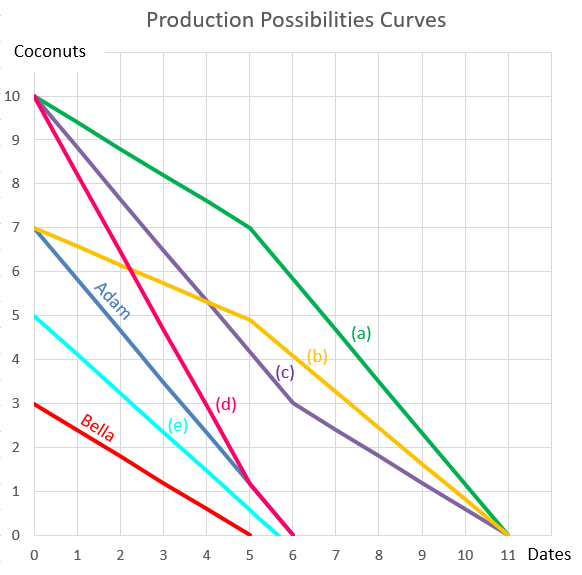# Fight Finance

#### CoursesTagsRandomAllRecentScores

A credit card offers an interest rate of 18% pa, compounding monthly.

Find the effective monthly rate, effective annual rate and the effective daily rate. Assume that there are 365 days in a year.

All answers are given in the same order:

$$r_\text{eff monthly} , r_\text{eff yearly} , r_\text{eff daily}$$

There are many ways to calculate a firm's free cash flow (FFCF), also called cash flow from assets (CFFA). Some include the annual interest tax shield in the cash flow and some do not.

Which of the below FFCF formulas include the interest tax shield in the cash flow?

$$(1) \quad FFCF=NI + Depr - CapEx -ΔNWC + IntExp$$ $$(2) \quad FFCF=NI + Depr - CapEx -ΔNWC + IntExp.(1-t_c)$$ $$(3) \quad FFCF=EBIT.(1-t_c )+ Depr- CapEx -ΔNWC+IntExp.t_c$$ $$(4) \quad FFCF=EBIT.(1-t_c) + Depr- CapEx -ΔNWC$$ $$(5) \quad FFCF=EBITDA.(1-t_c )+Depr.t_c- CapEx -ΔNWC+IntExp.t_c$$ $$(6) \quad FFCF=EBITDA.(1-t_c )+Depr.t_c- CapEx -ΔNWC$$ $$(7) \quad FFCF=EBIT-Tax + Depr - CapEx -ΔNWC$$ $$(8) \quad FFCF=EBIT-Tax + Depr - CapEx -ΔNWC-IntExp.t_c$$ $$(9) \quad FFCF=EBITDA-Tax - CapEx -ΔNWC$$ $$(10) \quad FFCF=EBITDA-Tax - CapEx -ΔNWC-IntExp.t_c$$

The formulas for net income (NI also called earnings), EBIT and EBITDA are given below. Assume that depreciation and amortisation are both represented by 'Depr' and that 'FC' represents fixed costs such as rent.

$$NI=(Rev - COGS - Depr - FC - IntExp).(1-t_c )$$ $$EBIT=Rev - COGS - FC-Depr$$ $$EBITDA=Rev - COGS - FC$$ $$Tax =(Rev - COGS - Depr - FC - IntExp).t_c= \dfrac{NI.t_c}{1-t_c}$$

A trader buys one crude oil European style put option contract on the CME expiring in one year with an exercise price of $44 per barrel for a price of$6.64. The crude oil spot price is $40.33. If the trader doesn’t close out her contract before maturity, then at maturity she will have the: You deposit money into a bank. Which of the following statements is NOT correct? You: A home loan company advertises an interest rate of 4.5% pa, payable monthly. Which of the following statements about the interest rate is NOT correct? The following cash flows are expected: • A perpetuity of yearly payments of$30, with the first payment in 5 years (first payment at t=5, which continues every year after that forever).
• One payment of $100 in 6 years and 3 months (t=6.25). What is the NPV of the cash flows if the discount rate is 10% given as an effective annual rate? A firm is about to conduct a 2-for-7 rights issue with a subscription price of$10 per share. They haven’t announced the capital raising to the market yet and the share price is currently $13 per share. Assume that every shareholder will exercise their rights, the cash raised will simply be put in the bank, and the rights issue is completed so quickly that the time value of money can be ignored. Disregard signalling, taxes and agency-related effects. Which of the following statements about the rights issue is NOT correct? After the rights issue is completed: Over the last year, a constant-dividend-paying stock's price fell, while it's future expected dividends and profit remained the same. Assume that: • Now is $t=0$, last year is $t=-1$ and next year is $t=1$; • The dividend is paid at the end of each year, the last dividend was just paid today $(C_0)$ and the next dividend will be paid next year $(C_1)$; • Markets are efficient and the dividend discount model is suitable for valuing the stock. Which of the following statements is NOT correct? The stock's: Adam and Bella are the only people on a remote island. Their production possibility curves are shown in the graph. Assuming that Adam and Bella cooperate according to the principles of comparative advantage, what will be their combined production possibilities curve?Last year, two friends Gear and Nogear invested in residential apartments. Each invested$1 million of their own money (their net wealth).

Apartments cost $1,000,000 last year and they earned net rents of$30,000 pa over the last year. Net rents are calculated as rent revenues less the costs of renting such as property maintenance, land tax and council rates. However, interest expense and personal income taxes are not deducted from net rents.

Gear and Nogear funded their purchases in different ways:

• Gear used $1,000,000 of her own money and borrowed$4,000,000 from the bank in the form of an interest-only loan with an interest rate of 5% pa to buy 5 apartments.
• Nogear used \$1,000,000 of his own money to buy one apartment. He has no mortgage loan on his property.

Both Gear and Nogear also work in high-paying jobs and are subject personal marginal tax rates of 45%.

Which of the below statements about the past year is NOT correct?